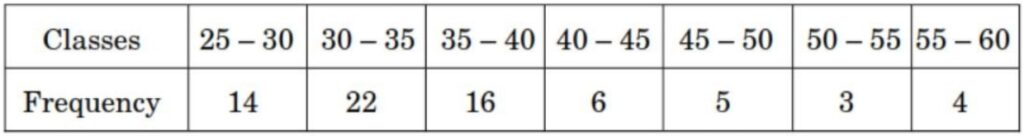Q) Find the mean of the following frequency distribution:Ans:

To calculate Mean value of the distribution, Let’s re-arrange the data with midpoint of each class, frequency, and multiply midpoint with frequency:We know that, mean of grouped data is given by:

Mean of grouped data =Therefore, Mean == 36.857

Hence, the mean value of the given distribution data is 36.86

Scroll to Top